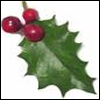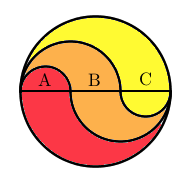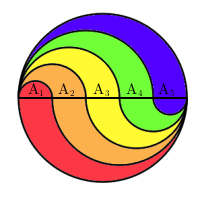#### You may also like### Giant Holly Leaf

Find the perimeter and area of a holly leaf that will not lie flat (it has negative curvature with 'circles' having circumference greater than 2πr).Given a square ABCD of sides 10 cm, and using the corners as centres, construct four quadrants with radius 10 cm each inside the square. The four arcs intersect at P, Q, R and S. Find the area enclosed by PQRS.### Get Cross

A white cross is placed symmetrically in a red disc with the central square of side length sqrt 2 and the arms of the cross of length 1 unit. What is the area of the disc still showing?

# Curvy Areas

##### Age 14 to 16Challenge Level
There are so many ways of solving this problem we can't write them all here. Abdulla sent us a clear method for finding the areas in the first circle. The key observation is that the curvy areas are all cut out by circles and semicircles.

To work out the area of the sections of the circle I first drew a diameter through the circle and labelled the areas above the diameter $A$, $B$, and $C$.I used $x$ for the radius of the smallest semicircle $A$. It has an area of
$A=\frac{1}{2}\pi x^2$

The area $B$ is made from a semicircle radius $2x$, minus the area $A$.
Then $B=\frac{1}{2}\pi (2x)^2 - \frac{1}{2}\pi x^2 = \frac{3}{2}\pi x^2$.

Area $C$ is a semicircle radius $3x$ minus a semicircle radius $2x$. Then $C=\frac{9}{2}\pi x^2-2\pi x^2=\frac{5}{2}\pi x^2$.

We can use symmetry to calculate the coloured areas.

The red shaded area is $A+C=3\pi x^2$.
The orange shaded area is $2B=3\pi x^2$.
The yellow shaded area is $A+C=3\pi x^2$.

It turns out they are all the same, and are $\frac{1}{3}$ of the total shaded area.

Niharika sent in a good idea for generalising. She guessed that the shaded areas are always the same proportion of the total area and managed to prove it!

Instead of using $A$, $B$, $C$ for the areas as in the $n=3$ case, we can call them $A_1$, $A_2$, ..., $A_n$. Let the big circle have radius $r$. Then $A_1$ is a semicircle radius $r/n$, and each $A_k$ is a semicircle radius $kr/n$ minus a semicircle radius $(k-1)r/n$.\begin{align}
A_k&=\frac{\pi}{2}\left(\frac{k^2r^2}{n^2}-\frac{(k-1)^2r^2}{n^2}\right)\\
&=\frac{\pi r^2}{2n^2}\left(2k-1\right)
\end{align}
And each shaded area coloured with the $k$th colour is made of a region area $A_k$ plus another area $A_{n+1-k}$. This is because the bottom half of the circle is the same as the top half, reflected and with the colours changed. Then the $k$th coloured shaded area is

\begin{align}
A_k+A_{n+1-k}&=\frac{\pi r^2}{2n^2}\left(2k-1\right)+\frac{\pi r^2}{2n^2}\left(2(n+1-k)-1\right)\\
&=\frac{\pi r^2}{2n^2}\left(2k-1 + 2n +2 -2k -1\right)\\
&=\frac{\pi r^2}{n}
\end{align}

Notice that this is $1/n$ of the total area $\pi r^2$ and it is the same for each of the $n$ colours (i.e. every $k$).

Fantastic work. Well done to everyone who found similar ways to generalise. Using algebra to deal with many different values at once, or unknown values is very important. In this question the calculations might be even easier if you work directly with fractions of area rather than with radii. Then there is no need to write down $\pi$.